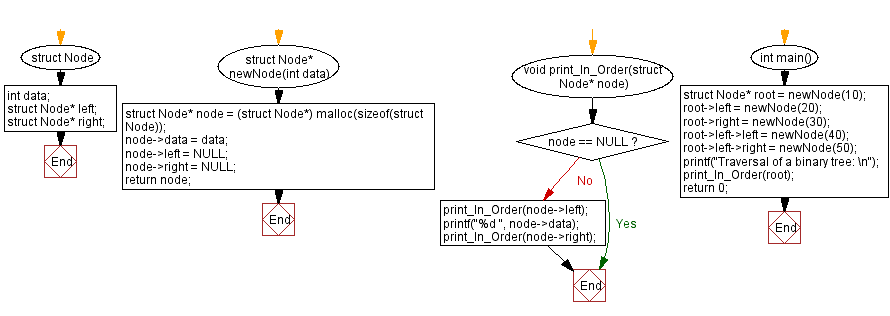﻿ C Program: Implement a binary tree using linked list representation

# C Exercises: Implement a binary tree using linked list representation

## C Singly Linked List : Exercise-27 with Solution

From Wikipedia -
In computer science, a binary tree is a k-ary k=2 tree data structure in which each node has at most two children, which are referred to as the left child and the right child. A recursive definition using just set theory notions is that a (non-empty) binary tree is a tuple (L, S, R), where L and R are binary trees or the empty set and S is a singleton set containing the root. Some authors allow the binary tree to be the empty set as well.

Write a C program to implement a binary tree using linked list representation.

The function uses the "Right-Root-Left" traversal order, which means it first traverses the right subtree, then the root node, and finally the left subtree.

Sample Solution:

C Code:

``````#include<stdio.h>
#include <stdlib.h>

struct Node {
int data;
struct Node* left;
struct Node* right;
};

struct Node* newNode(int data) {
struct Node* node = (struct Node*) malloc(sizeof(struct Node));
node->data = data;
node->left = NULL;
node->right = NULL;
return node;
}

void print_In_Order(struct Node* node) {
if (node == NULL) return;
print_In_Order(node->left);
printf("%d ", node->data);
print_In_Order(node->right);
}

int main()
{
struct Node* root = newNode(10);
root->left = newNode(20);
root->right = newNode(30);
root->left->left = newNode(40);
root->left->right = newNode(50);
printf("Traversal of a binary tree: \n");
print_In_Order(root);
return 0;
}
```
```

Sample Output:

```Traversal of a binary tree:
40 20 50 10 30
```

Flowchart :C Programming Code Editor:

What is the difficulty level of this exercise?

﻿